+

# Converting Units

Author: Sophia Tutorial
##### Description:

Use simple conversion factors to convert units in a given scenario.

(more)

Sophia’s self-paced online courses are a great way to save time and money as you earn credits eligible for transfer to many different colleges and universities.*

No credit card required

37 Sophia partners guarantee credit transfer.

299 Institutions have accepted or given pre-approval for credit transfer.

* The American Council on Education's College Credit Recommendation Service (ACE Credit®) has evaluated and recommended college credit for 32 of Sophia’s online courses. Many different colleges and universities consider ACE CREDIT recommendations in determining the applicability to their course and degree programs.

Tutorial
what's covered
1. Introduction to Converting Units
2. Simple Unit Conversion
3. Multi-step Unit Conversion
4. Converting Units of Area and Volume

# 1. Introduction to Converting Units

Unit conversion is a simple process to overlook, but doing so can have dramatic effects on the results of any project we may be working on. For example, suppose someone told us that a machine needs to be able to handle a 50,000 N load. If we were in a country that uses the metric system, that may make perfect sense to us. If we are in the United States, where English units are used, that may not make any sense at all. The need then arises to convert 50,000 N into pounds (lbs.), so that we can more easily understand the quantities we are working with. Unit conversion allows us to express 50,000 N as roughly 11,240 lbs.,or 5.62 tons. While the numbers and units are different, the actual quantity they represent is the same.

big idea
Notice that each of these values has different units, but they represent the same amount of force. That is why unit conversion is important. It allows us to represent quantities in terms of measurements we understand or need to work with.

Lets look at how to do some unit conversion.

# 2. Simple Unit Conversion

In order to convert units, we first need to understand something called a conversion factor, which is basically a fraction equaling 1 that relates two different units. For example, suppose we have 160 cups of water, and we want to determine how many gallons of water this is equal to. Before we make any calculations, we might recall that there are 16 cups in one gallon of water. This knowledge will help us determine what our conversion factor will be.

So how do we actually begin converting?

1. List the value you are given.
2. Determine the conversion factor.
3. Multiply the conversion factor to the given value, making sure that the units we start with cancel or and the unit we are looking for will be left.
4. Simplify as needed.

For the problem involving cups to water, this would look like the following: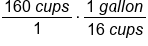Conversion factor: 1 gallon=16 cups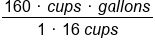Multiplying across numerators and denominators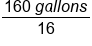Units of cups cancel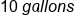Our Solution

Let’s look at a couple more examples.

brainstorm
Suppose we wanted to convert 7200 seconds into hours, and 2 miles into feet. Try doing these calculations on your own, and then come back to check the solutions.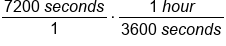Conversion factor: 1 hour=3600 seconds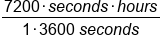Multiplying across numerators and denominators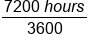Units of seconds cancel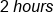Our Solution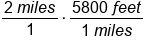Conversion factor: 5280 feet=1 mile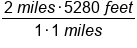Multiply numerators and denominators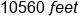Our Solution

# 3. Multi-Step Unit Conversion

In the above example of converting seconds to hours, suppose we did not know a conversion factor between hours and seconds. Do you think we can make the conversion?

Of course! In cases such as this, we may wish to use multiple conversion factors to help us make a conversion. For example, we may know that there are 60 seconds in 1 minute, and 60 minutes in 1 hour.

Therefore, we can do the following: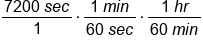Conversion factors: 1 min=60 sec; 1 hr=60 min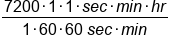Multiply numerators and denominators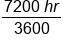Units of seconds and minutes cancel, leaving hoursOur Solution

brainstorm
Try your hand at converting 3 meters into inches. Note that there are 2.54 centimeters in 1 inch, and 100 centimeters in 1 meter.

To solve this problem, we can implement the conversion factors 2.54 centimeter = 1 inch and 100 centimeter = 1 meter, and string the conversion factors together.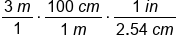Conversion factors:1 m=100 cm; 1 in=2.54 cm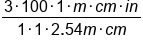Multiply numerators and denominators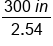Units of meters and centimeters cancel, leaving inches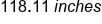Our Solution

# 4. Converting Units of Area and Volume

Sometimes when converting units, we may need to convert between squared units (area) or cubed units (volume). In these instances, we follow the same process as before, but we have to be careful with our conversion factors. Let's look at some examples.

Suppose we are told that we need to convert 200 square feet in to square inches. How would we go about doing this calculation?

hint
Before we begin the conversion, we need to determine if we are using the correct conversion factor. Here, we may be tempted to use 12 inches in 1 foot, making the conversion factor (12 inches / 1 foot). However, we would be incorrect when making this calculation. In truth, there are 12 inches • 12 inches, or 144 square inches in 1 square foot.

Therefore, our conversion factor needs to be (144 square inches / 1 square foot). Going through the calculations, we would do the following: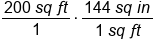Conversion factor: 1 sq ft= 144 sq in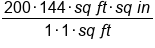Multiply numerators and denominators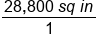Units of square feet cancel, leaving square inches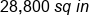Our Solution

The key idea here is that we have to match squared units with squared units when choosing the conversion factor.

Let’s look at another example.

Suppose you want to convert 5 cubic feet to liters. How would you make this conversion given that there are approximately 30.48 centimeters in 1 foot in 1 cubic feet and 0.001 liters in 1 cubic centimeter?

Here, we have to first determine a conversion factor between cubic feet and liters. Right now, we do not have a conversion factor relating the two, however we do know how many centimeter are in 1 foot. Therefore, if we take the cube of 30.48 centimeters, we would know how many cubic centimeters there are in 1 cubic feet. With this knowledge we can use the other conversion factor we can given to convert between cubic centimeters to liters.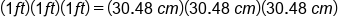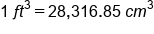Using this conversion factor, we can now being making our conversion.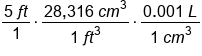Conversion factors factors for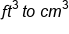and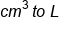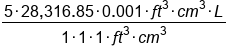Multiply numerators and denominators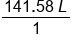Units of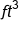and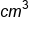cancel, leaving L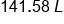Our Solution, rounded to the hundredths place

When working with volume conversion, you must make sure your conversion factor is converting between cubic units.

summary
As an introduction to converting units, we learned that unit conversion allows us to express measurements with different units. They are the same measurement, but with different units. We can use simple unit conversion for any type of measurement such as length, time, area, volume, or rate, such as miles per hour. Some cases require multi-step unit conversions, such as hours to minutes to seconds. When converting units of area and volume, you're converting with square units or cubic units. Square units requires you to square the linear conversion, while cubic units requires you to cube the linear conversion.

Terms to Know
Conversion Factor

A fraction equal to one that is multiplied by a quantity to convert it into an equivalent quantity in different units.

Rating